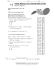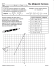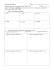10.3 Coordinate Proof Using Distance with Segments and Triangles

Transcription

10.3 Coordinate Proof Using Distance with Segments and Triangles
DO NOT EDIT--Changes must be made through "File info"
CorrectionKey=NL-A;CA-A
Name
Class
Date
10.3 Coordinate Proof Using
Distance with Segments
and Triangles
Resource
Locker
Essential Question: How do you write a coordinate proof?
Deriving the Distance Formula
and the Midpoint Formula
Explore
Complete the following steps to derive the Distance Formula and the Midpoint Formula.
A
To derive the Distance Formula, start with points J and K as shown in the figure.
K (x2, y2)
y
Given: J(x 1, y 1) and K(x 2, y 2) with x 1 ≠ x 2 and y 1 ≠ y 2
Prove: JK =
――――――――
√(x 2 - x 1) 2 + (y 2 - y 1) 2
L
x
J (x1, y1)
_
Locate point L so that JK is the hypotenuse of right triangle JKL. What are the
coordinates of L?
(x 2, y 1)
B
Find JL and LK.
JL = x 2 - x 1, LK = y 2 - y 1
© Houghton Mifflin Harcourt Publishing Company
C
By the Pythagorean Theorem, JK 2 = JL 2 + LK 2. Use this to find JK. Explain your steps.
JK = (x 2 - x 1) 2 + (y 2 - y 1) 2 by substitution.
2
Taking the square root of both sides shows that JK =
D
――――――――
√(x 2 - x 1) 2 + (y 2 - y 1) 2 .
To derive the Midpoint Formula, start with points A and B as shown in
the figure.
Given: A(x 1, y 1) and B(x 2, y 2)
_
y1 + y2
x1 + x2 _
,
Prove: The midpoint of AB is M _
.
2
2
(
)
y
B (x2, y2)
y2
M
y1
A (x1, y1)
x1
x
x2
What is the horizontal distance from point A to point B? What is the
vertical distance from point A to point B?
horizontal distance: x 2 - x 1; vertical distance: y 2 - y 1
Module 10
GE_MNLESE385795_U3M10L3.indd 521
521
Lesson 3
02/04/14 5:46 AM
DO NOT EDIT--Changes must be made through "File info"
CorrectionKey=NL-A;CA-A
E
F
DO NOT
Correcti
The horizontal and vertical distances from A to M must be half
these distances.
x2 - x1
2
What is the horizontal distance from point A to point M?
y2 - y1
2
What is the vertical distance from point A to point M?
_
_
To find the coordinates of point M, add the distances from Step E
to the x- and y-coordinates of point A and simplify.
y
B (x2, y2)
y2
M
y1
x
x1 A (x , y ) x2
1 1
2x
x2 - x1
2x 1 + x 2 - x 1 _
x + x2
x2 - x1 _
x-coordinate of point M: x 1 + _
= 1+_
= __
= 1
2
2
2
2
2
2y 1
y2 - y1
2y 1 + y 2 - y 1
y1 + y2
y2 - y1
=
+
=
=
y1 +
2
2
2
2
2
y-coordinate of point M:
_ _ _ __ _
Reflect
1.
In the proof of the Distance Formula, why do you assume that x 1 ≠ x 2 and y 1 ≠ y 2?
If x 1 = x 2 or y 1 = y 2, the segment is vertical or horizontal and it is not possible to form a
right triangle as in the figure.
2.
Does the Distance Formula still apply if x 1 = x 2 or y 1 = y 2? Explain.
Yes; if x 1 = x 2, the formula simplifies to JK = ⎜y 2 - y 1⎟, and if y 1 = y 2, the formula simplifies
to JK = ⎜x 2 - x 1⎟.
3.
Does the Midpoint Formula still apply if x 1 = x 2 or y 1 = y 2? Explain.
y1 + y2
, and if y 1 = y 2, the formula simplifies
Yes; if x 1 = x 2, the formula simplifies to M x 1,
2
x1 + x2
, y1 .
to M
2
(_ )
(
_)
© Houghton Mifflin Harcourt Publishing Company
Module 10
GE_MNLESE385795_U3M10L3.indd 522
522
Lesson 3
3/22/14 10:04 AM
DO NOT EDIT--Changes must be made through "File info"
CorrectionKey=NL-A;CA-A
Explain 1
Positioning a Triangle on the
Coordinate Plane
A coordinate proof is a style of proof that uses coordinate geometry and algebra. The first step
of a coordinate proof is to position the given figure in the plane. You can use any position, but
some strategies can make the steps of the proof simpler.
Strategies for Positioning Figures in the Coordinate Plane
• Use the origin as a vertex, keeping the figure in Quadrant I.
• Center the figure at the origin.
• Center a side of the figure at the origin.
• Use one or both axes as sides of the figure.
Example 1 Write each coordinate proof.

_
Given: ∠B is a right angle in △ABC. D is the midpoint of AC.
Prove: The area of △DBC is one half the area of △ABC.
Step 1 Assign coordinates to each vertex. Since you will use the
Midpoint Formula to find the coordinates of D, use multiples
of 2 for the leg lengths.
The coordinates of A are (0, 2j).
The coordinates of B are (0, 0).
The coordinates of C are (2n, 0).
Step 2 Position the figure on the coordinate plane.
A (0, 2j)
y
Step 3 Write a coordinate proof.
D
△ABC is a right triangle with height 2j and base 2n.
© Houghton Mifflin Harcourt Publishing Company
area of △ABC = _12bh
= _12(2n)(2j)
= 2nj square units
x
B (0, 0)
(
C (2n, 0)
)
2j + 0
0 + 2n _
,
By the Midpoint Formula, the coordinates of D = _
= (n, j).
2
2
The height of △DBC is j units, and the base is 2n units.
area of △DBC = _12bh
= _12(2n)(j)
= nj square units
Since nj = _12 (2nj), the area of △DBC is one half the area of △ABC.
Module10
GE_MNLESE385795_U3M10L3.indd 523
523
Lesson3
3/22/14 10:04 AM
DO NOT EDIT--Changes must be made through "File info"
CorrectionKey=NL-A;CA-A
B
DO NOT
Correcti
_
Given: ∠B is a right angle in △ABC. D is the midpoint of AC.
Prove: The area of △ADB is one half the area of △ABC.
Assign coordinates and position the figure as in Example 1A.
△ABC is a right triangle with height 2j and base 2n .
D
x
area of △ABC = _12bh
= _12 2n ⋅ 2j
= 2nj square units
y
A (0, 2j)
B (0, 0)
(
) (
0 + 2n
2j + 0
_______ =
By the Midpoint Formula, the coordinates of D = _______
,
2
2
The height of △ADB is n units, and the base is 2j units.
n ,
C (2n, 0)
j
).
area of △ADB = _12bh = _12 2j ⋅ n = jn square units
_1
Since jn = 2 (2 nj) , the area of △ADB is one half the area of △ABC.
Reflect
4.
Why is it possible to position △ABC so that two of its sides lie on the axes of the
coordinate plane?
It is given that △ABC has a right angle at ∠B. The axes of the coordinate plane intersect at a
right angle, so if vertex B is at the origin, then two sides of the triangle will lie on the axes.
Position the given triangle on the coordinate plane. Then show that the result about
areas from Example 1 holds for the triangle.
5.
1
(2)(4) = 4 units 2
The height of △ABC is 4 units, and the base is 2 units. area of △ABC = __
2
¯, are
The coordinates of D, the midpoint of AC
+2 4
+0
, ____
= (1, 2).
(0____
2
2 )
1
(2)(2) = 2 units 2
The height of △DBC is 2 units, and the base is 2 units. area of △DBC = __
2
1
(4), the area of △DBC is one half the area of △ABC.
Since 2 = __
2
Module 10
GE_MNLESE385795_U3M10L3.indd 524
524
© Houghton Mifflin Harcourt Publishing Company
A right triangle, △ABC, with legs of length 2 units and 4 units
Possible answer: Let the coordinates of the vertices be A(0, 4), B(0, 0), and C(2, 0).
Lesson 3
3/22/14 10:04 AM
DO NOT EDIT--Changes must be made through "File info"
CorrectionKey=NL-A;CA-A
6.
A right triangle, △ABC, with both legs of length 8 units
Possible answer: Let the coordinates of the vertices be A(0, 8), B(0, 0), and C(8, 0).
1
(8)(8) = 32units 2
The height of △ABC is 8 units, and the base is 8 units. area of △ABC = __
2
(
)
+8 8
+0
¯, are 0____
The coordinates of D, the midpoint of AC
, ____
= (4, 4).
2
2
1 ( )( )
The height of △DBC is 4 units, and the base is 8 units. area of △DBC = _
8 4 = 16 units2
2
1( )
Since 16 = _
32 , the area of△DBC is one half the area of △ABC.
2
Explain 2
Proving the Triangle Midsegment Theorem
In Module 8, you learned that the Triangle Midsegment Theorem states that a midsegment
of a triangle is parallel to the third side of the triangle and is half as long as the third side.
You can now use a coordinate proof to show that the theorem is true.
Example 2
Prove the Triangle Midsegment Theorem.
_
Given: XY is a midsegment of △PQR.
_ _
Prove: XY ∥ PQ and XY = _12PQ
P
X
Place △PQR so that one vertex is at the origin. For convenience, assign
vertex P the coordinates (2a, 2b) and assign vertex Q the vertices (2c, 2d).
)
y
P (2a, 2b)
0 + 2b
0 + 2a _
,
The coordinates of X are X _
= X( a,b ).
2
2
(
0
+
0
2c
+
2d
)
Y
R
Use the Midpoint Formula to find the coordinates of X and Y.
(
(
X
)
Q (2c, 2d)
, ___________
=Y c , d .
The coordinates of Y are Y ___________
2
2
R (0, 0)
_
_
Find the slope of PQand XY.
d - b
d - b
_ y2 - y1
_ _
y2 - y1
2d - 2b = ___________ ; slope of XY
_
___________
=
=
slope of PQ = _
=
x2 - x1
x2 - x1
2c - 2a
© Houghton Mifflin Harcourt Publishing Company
c
-
Q
a
c
-
Y
x
a
_ _
Therefore, PQ ǁ XY sincethe slopes are the same.
Use the Distance Formula to find PQ and XY.
____
PQ = √(x 2 - x 1) 2 + ( y 2 - y 1 ) 2
= =
――――――――――――
√
4 ⋅ (c - a) 2 + 4 ⋅ ( d - b ) 2
――
√
___
4 ⋅ √(c - a) 2 + (d - b) 2
____
XY = √ (x 2 - x 1) 2 + (y 2 - y 1) 2
____
= √(2c - 2a) + (2d - 2b)
2
=
=
=
2
―――――――――
√4
⋅ (c - a) 2 + (d - b)
2
___
2 √(c - a) 2 + (d - b)
2
_____
√(
) (
2
c - a
+
d - b
)
2
1
This shows that XY = _____ PQ.
2
Module10
GE_MNLESE385795_U3M10L3.indd 525
525
Lesson3
3/22/14 10:04 AM
DO NOT EDIT--Changes must be made through "File info"
CorrectionKey=NL-A;CA-A
DO NOT
Correcti
Reflect
7.
Discussion Why is it more convenient to assign vertex P the coordinates (2a, 2b) and vertex Q the
coordinates (2c, 2d) rather than using the coordinates (a, b) and (c, d)?
¯. Assigning
¯ and RQ
The proof requires finding the coordinates of the midpoints of RP
coordinates that are multiples of 2 eliminates the need for fractions in the coordinates of
the midpoints.
Explain 3
Proving the Concurrency of Medians Theorem
You used the Concurrency of Medians Theorem in Module 8 and proved it in Module 9.
Now you will prove the theorem again, this time using coordinate methods.
Example 3
Prove the Concurrency of Medians Theorem.
Q
N
_ _
_
Given: △PQR with medians PL, QM, and RN
_ _
_
Prove: PL, QM, and RN are concurrent.
P
L
M
R
Place △PQR so that vertex R is at the origin. Also, place the triangle so that point N lies
on the y-axis. For convenience, assign point N the vertices (0, 6a). (The factor of 6 will
result in easier calculations later.)
y
Q
N (0, 6a)
P
x
_
Since N is the midpoint of PQ, assign coordinates to P and Q as follows.
y
The horizontal distance from N to P must be the same
as the horizontal distance from N to Q. Let this distance be 2b.
Then the x-coordinate of point P is -2b and the x-coordinate
of point Q is
2b .
Q ( 2b , 6a + 2c )
N (0, 6a)
P ( -2b , 6a - 2c )
The vertical distance from N to P must be the same as the
vertical distance from N to Q. Let this distance be 2c.
Then the y-coordinate of point P is 6a - 2c and the y-coordinate
of point Q is 6a + 2c .
© Houghton Mifflin Harcourt Publishing Company
R (0, 0)
x
R (0, 0)
Complete the figure by writing the coordinates of points P and Q.
Module10
GE_MNLESE385795_U3M10L3.indd 526
526
Lesson3
3/22/14 10:04 AM
DO NOT EDIT--Changes must be made through "File info"
CorrectionKey=NL-A;CA-A
Now use the Midpoint Formula to find the coordinates of L and M.
(
(
)
(b
0 + 6a + 2c
0 + 2b
_
The midpoint of RQ is L __ , __ = L
2
2
)
,
3a + c
(
)
.
0 + -2b
0 + 6a - 2c
_
3a - c
The midpoint of RP is M __ , __ = M -b ,
2
2
)
.
y
Complete the figure by writing the coordinates of points L and M.
‹ ›
−
To complete the proof, write the equation of QM and use
the equation to find the coordinates
of
_
_point C, which is the
intersection of the medians QM and RN. Then show that
‹ ›
−
point C lies on PL .
Q ( 2b , 6a + 2c )
N (0, 6a)
P ( -2b , 6a - 2c )
C
L ( b , 3a + c )
M ( -b , 3a - c )
‹ ›
−
Write the equation of QM using point-slope form.
x
R (0, 0)
a + c
3 a +3 c
‹ › ( 6a + 2c ) - ( 3a - c )
−
The slope of QM is __ = __ = __.
2b - (-b)
3 b
b
‹ ›
−
Use the coordinates of point Q for the point on QM .
)
(
a + c
‹ ›
−
Therefore, the equation of QM is y - 6a + 2c = __ ⋅ x - 2b .
b
Since point C lies on the y-axis, the x-coordinate of point C is 0. To find the y-coordinate of C,
‹ ›
−
substitute x = 0 in the equation of QM and solve for y.
a + c
Substitute x = 0.
y - 6a + 2c = __ ⋅ 0 - 2b
(
© Houghton Mifflin Harcourt Publishing Company
b
Simplify the right side of the equation.
y - 6a + 2c = -2
Distributive property
y - 6a + 2c = -2 a - 2 c
Add 6a + 2c to each side and simplify.
(0
So, the coordinates of point C are C
)
)
a+c
y = 4a
, 4a .
‹ ›
−
Now write the equation of PL using point-slope form.
3 a -3 c
a - c
‹ › ( 6a - 2c ) - (3a + c)
−
The slope of PL is __ = __ = __.
-2b - b
-3 b
- b
‹ ›
−
Use the coordinates of point P for the point on PL .
(
)
a - c
‹ ›
−
Therefore, the equation of PL is y - 6a - 2c = __ ⋅ x + 2b .
- b
Module 10
GE_MNLESE385795_U3M10L3.indd 527
527
Lesson 3
3/22/14 10:04 AM
DO NOT EDIT--Changes must be made through "File info"
CorrectionKey=NL-A;CA-A
DO NOT
Correcti
‹ ›
−
Finally, show that point C lies on PL . To do so, show that when x = 0 in the
‹ ›
−
equationfor PL , y = 4a.
(
Substitute x = 0.
a - c
y - 6a - 2c = __ ⋅ 0 + 2b
- b
Simplify right side of equation.
y - 6a - 2c = -2 a + 2 c
Add 6a - 2c to each side and simplify.
)
y = 4a
Reflect
8.
A student claims that the averages of the x-coordinates and of the y-coordinates of the
vertices of the triangle are x- and y-coordinates of the point of concurrency, C. Does the
coordinate proof of the Concurrency of Medians Theorem support the claim? Explain.
Yes. The x-coordinates of the vertices of the triangle are −2b, 0, and 2b. The average of
these coordinates is 0. The y-coordinates of the vertices of the triangle are 6a - 2c, 0, and
6a + 2c. The average of these coordinates is 4a. These averages match the coordinates of
point C, (0, 4a).
Using Triangles on the Coordinate Plane
Explain 4
Example 4 Write each proof.

Given: A(2, 3), B(5, −1), C(1, 0), D(-4, −1), E(0, 2), F(−1, −2)
Prove: ∠ABC ≅ ∠DEF
Step 1 Plot the points on a coordinate plane.
y A
E
x
___
_
D
___
-2
0 C
F
2
B
_
2
2
2
2
AB = √(5 - 2) + (-1 - 3) = √ 25 = 5; BC = √ (1 - 5) + 0 - (-1) = √17;
___
AC = √ (1 - 2) + (0 - 3) =
2
2
_
√ 10;
____
____
DE = √(0 - (-4)) + (2 - (-1)) = √25 = 5;
_
2
2
_____
EF = √(-1 - 0) + (-2 - 2) = √1 +16 = √17; DF = √(-1 - (-4)) + (-2 - (-1))
2
_
2
_
_
2
2
_
= √9 +1 = √10
_ _ _ _
_ _
So, AB ≅ DE, BC ≅ EF, and AC ≅ DF. Therefore, △ABC ≅ △DEF by the
SSS Triangle Congruence Theorem and ∠ABC ≅ ∠DEF by CPCTC.
Module10
GE_MNLESE385795_U3M10L3.indd 528
528
© Houghton Mifflin Harcourt Publishing Company
Step 2 Use the Distance Formula to find the length of each side of
each triangle.
Lesson3
3/22/14 10:04 AM
DO NOT EDIT--Changes must be made through "File info"
CorrectionKey=NL-A;CA-A
B
_
Given: J(-4, 1), K(0, 5), L(3, 1), M(−1, −3), R is the midpoint of JK, S is the midpoint
_
of LM.
Prove: ∠JSK ≅ ∠LRM
Step 1 Plot the points on a coordinate plane.
y K
4
R
(
(
)
)
(
)
1 + 5
-4 + 0
R __ , __ = R -2 3
,
2
2
(
-4
)
1 + -3
3 + -1
S __ , __ = S 1 -1
2
2
,
Step 3 Use the Distance Formula to find the length of each side of each triangle.
____
JK = √(0 - (-4)) + (5 - 1) = √16 + 16 = √32
2
__
2
____
KS =
JS =
√( 1
√( 1
) (
) =√ 1
2
- 0 + -1 - 5
) (
_____
- (-4) + -1 - 1
____
2
__
=
__
√( -2
2
) (3
)
_____
MR =
© Houghton Mifflin Harcourt Publishing Company
LR =
2
- (-1) +
- (-3)
____
√( -2 - 3) + ( 3
2
2
S
2
4
M
-4
4
_
=
√ 29
_
__
=
√
) = √ 25 +
-1
2
-2
√ 37
LM = √(-1 - 3) + (-3 - 1) = √16 + 16 = √32
2
0
x
_
+ 36 =
) √ 25 +
2
-2
L
_
__
2
2
J
Step 2 Use the Midpoint Formula to find the coordinates of R and S.
1 + 36 =
__
4 =
_
_
¯
¯ _ ¯
So, JK ≅ LM , KS ≅ MR , and JS ≅ LR . Therefore, △JKS ≅
_
_
√ 37
√ 29
△LMR by the
SSS Triangle Congruence Theorem and ∠JSK ≅ ∠LRM
corresponding parts of congruent triangles are congruent .
since
Reflect
9.
In Part B, what other pairs of angles can you prove to be congruent? Why?
∠JKS ≅ ∠LMR and ∠KJS ≅ ∠MLR because these are corresponding parts of congruent
triangles.
Module 10
GE_MNLESE385795_U3M10L3.indd 529
529
Lesson 3
3/22/14 10:04 AM
DO NOT EDIT--Changes must be made through "File info"
CorrectionKey=NL-A;CA-A
DO NOT
Correcti
Write each proof.
10. Given: A(-4, −2), B(−3, 2), C(−1, 3), D(-5, 0), E(−1, −1), F(0, −3)
Prove: ∠BCA ≅ ∠EFD
―
―
―
―
―
By the Distance Formula, AB = √17 , BC = √5 , AC = √34 , DE = √17 , EF = √5 ,
―
¯ ≅ EF
¯ ≅ DF
¯ ≅ DE
¯, BC
¯. Therefore, △ABC ≅ △DEF by the
¯, and AC
DF = √34 . So, AB
SSS Triangle Congruence Theorem and ∠BCA ≅ ∠EFD since corresponding parts of
congruent triangles are congruent.
_
_
11. Given: P(−3, 5), Q(−1, −1), R(4, 5), S(2, −1), M is the midpoint of PQ, N is the midpoint of RS.
Prove: ∠PQN ≅ ∠RSM
By the Midpoint Formula, the coordinates of M are M
the coordinates of N are N
(____, _______) = N(3,2).
4 + 2 5 + (-1)
2
2
―
(________, _______) = M(-2, 2) and
-3 + (-1) 5 + (-1)
2
2
―
―
―
By the Distance Formula, PQ = √40 , QN = 5, PN = √45 , RS = √40 , SM = 5, RM = √45 .
¯ ≅ RS
¯ ≅ SM
¯, QN
¯, and PN
¯ ≅ RM
¯. Therefore, △PQN ≅ △RSM by the SSS Triangle
So, PQ
Congruence Theorem and ∠PQN ≅ ∠RSM since corresponding parts of congruent triangles
are congruent.
Elaborate
12. When you write a coordinate proof, why might you assign 2p as a coordinate rather than p?
If the proof includes finding the midpoint of a segment, assigning the coordinate 2p might
make it possible to avoid fractions in the coordinates of the midpoint.
geometry and algebra to draw conclusions. Coordinate proofs often involve the Distance
Formula, the Midpoint Formula, and/or the Slope Formula.
Module 10
GE_MNLESE385795_U3M10L3.indd 530
530
© Houghton Mifflin Harcourt Publishing Company
13. Essential Question Check-In What makes a coordinate proof different from the other types of proofs
you have written so far?
A coordinate proof depends upon assigning coordinates to a figure and using coordinate
Lesson 3
3/22/14 10:04 AM

HSM12CC_GM_06_09_CM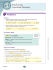Page 1 Proving a triangle is a right triangle Method 1: Show two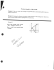Coordinate Geometry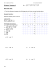Algebra with shapes How to . . .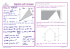Reteach Sec 1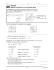5.1 Midsegment Theorem and Coordinate Proof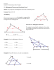Final Review Finally - Mr. Mayer AfterMath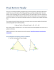Solve the problems below to answer the riddle: